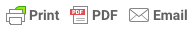### What is central limit theorem in statistics?

By Saul McLeod, published Nov 25, 2019

The central limit theorem states that the sampling distribution of the mean approaches a normal distribution, as the sample size increases. This fact holds especially true for sample sizes over 30.

Therefore, as a sample size increases, the sample mean and standard deviation will be closer in value to the population mean μ and standard deviation σ .###### Why is central limit theorem important?

The central limit theorem tells us that no matter what the distribution of the population is, the shape of the sampling distribution will approach normality as the sample size (N) increases.

This is useful, as the research never knows which mean in the sampling distribution is the same as the population mean, but by selecting many random samples from a population the sample means will cluster together, allowing the research to make a very good estimate of the population mean.

Thus, as the sample size (N) increases the sampling error will decrease.

### Summary

• As the sample size increases, the distribution of frequencies approximates a bell-shaped curved (i.e. normal distribution curve).

• Sample size equal to or greater than 30 are required for the central limit theorem to hold true.

• A sufficiently large sample can predict the parameters of a population such as the mean and standard deviation.

McLeod, S. A. (2019, Nov 25). What is central limit theorem in statistics? Simply psychology: https://www.simplypsychology.org/central-limit-theorem.html